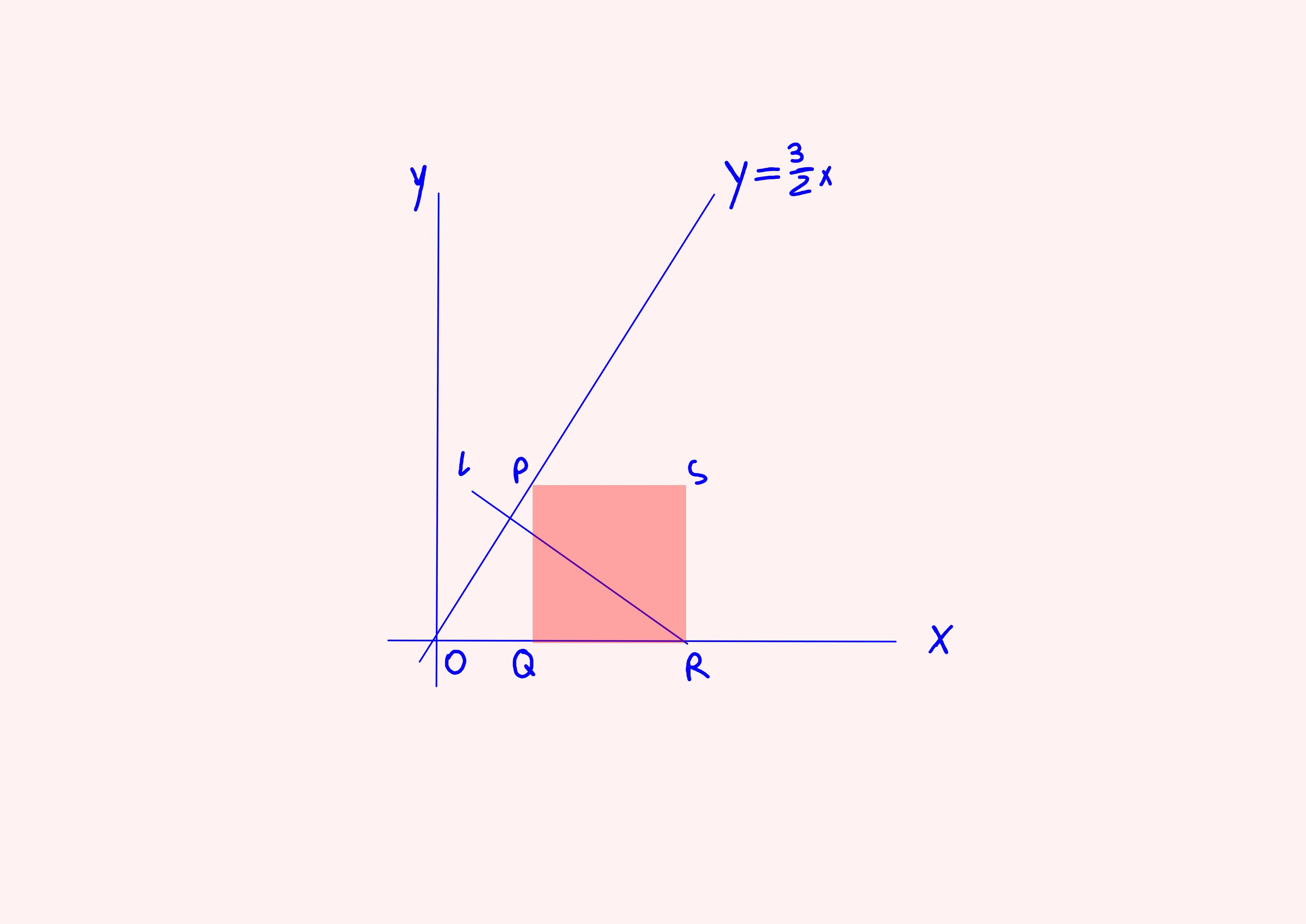# In the diagram to the right, point P lies on the line y=3/(2)x and is one vertex of square PQRS. Point R has coordinates (5,0). a. Find the coordinates of point P. b. If point R lies on line l, and line I divides quadrilateral PQRS into two regions of equal area, find the equation for line l.aanpalendmw 2022-07-16 Answered
In the diagram to the right, point $P$ lies on the line $y=\frac{3}{2}x$ and is one vertex of square $PQRS$. Point $R$ has coordinates $\left(5,0\right)$.
a. Find the coordinates of point $P$.
b. If point $R$ lies on line $l$, and line $I$ divides quadrilateral $PQRS$ into two regions of equal area, find the equation for line $l$.You can still ask an expert for help

• Live experts 24/7
• Questions are typically answered in as fast as 30 minutes
• Personalized clear answers

Solve your problem for the price of one coffee

• Math expert for every subject
• Pay only if we can solve itBubbinis4
Hint: The first big step to solving this problem is to figure out the $x$ and $y$ coordinate of $P$. Notice that there are two distinct constraints on $P$: it must lie on the line $y=\frac{3}{2}x$, and it must be the corner of a square. For me, these problems are easier to think about if they are written entirely in equations. So my advice would be to figure out "what does it mean to be on the corner of that square?"
Keep in mind that you already know the coordinate of one corner, and you also know a few things about squares.
For the second part, you should notice that it only takes two points to determine a line. If you choose a second point at random, this will be a rather difficult problem.

We have step-by-step solutions for your answer!